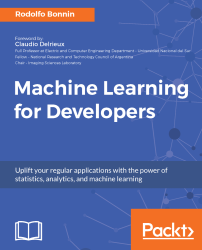•#### Machine Learning for Developers#### Overview of this book

Most of us have heard about the term Machine Learning, but surprisingly the question frequently asked by developers across the globe is, “How do I get started in Machine Learning?”. One reason could be attributed to the vastness of the subject area because people often get overwhelmed by the abstractness of ML and terms such as regression, supervised learning, probability density function, and so on. This book is a systematic guide teaching you how to implement various Machine Learning techniques and their day-to-day application and development. You will start with the very basics of data and mathematical models in easy-to-follow language that you are familiar with; you will feel at home while implementing the examples. The book will introduce you to various libraries and frameworks used in the world of Machine Learning, and then, without wasting any time, you will get to the point and implement Regression, Clustering, classification, Neural networks, and more with fun examples. As you get to grips with the techniques, you’ll learn to implement those concepts to solve real-world scenarios for ML applications such as image analysis, Natural Language processing, and anomaly detections of time series data. By the end of the book, you will have learned various ML techniques to develop more efficient and intelligent applications.
PrefaceFree Chapter
Introduction - Machine Learning and Statistical ScienceThe Learning ProcessClusteringLinear and Logistic RegressionNeural NetworksConvolutional Neural NetworksRecurrent Neural NetworksRecent Models and DevelopmentsSoftware Installation and Configuration# Deploying a deep neural network with Keras

In this exercise, we will generate an instance of the previously described Inception model, provided by the Keras application library. First of all, we will import all the required libraries, including the Keras model handling, the image preprocessing library, the gradient descent used to optimize the variables, and several Inception utilities. Additionally, we will use OpenCV libraries to adjust the new input images, and the common NumPy and matplotlib libraries:

```from keras.models import Model
from keras.preprocessing import image
from keras.optimizers import SGD
from keras.applications.inception_v3 import InceptionV3, decode_predictions, preprocess_input

import matplotlib.pyplot as plt
import numpy as np
import cv2Using TensorFlow backend.```

Keras makes it really simple to load a model. You just have to invoke a new instance of the...